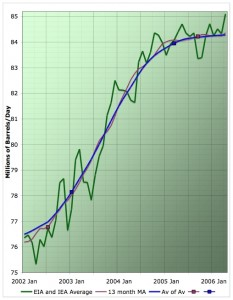# Trend Technician

Just another WordPress weblog

## Technical Analysis Basics: Moving Averages

February 3, 2010 | No Comments | Course

The most commonly used indicator by far is the moving average. Its value is easy to understand intuitively and one of the least controversial indicators. Almost any trader will agree to the value of moving averages.

## Moving Average Basics

A moving average represents the average value of an issue over a trailing window.  All moving averages are designated in terms of a time span for how many days of trailing data should be included in the average.  For example, 10 day, 50 day and 200 day moving averages are commonly used.  There are however several ways to calculate this average.  The two most common are the simple moving average and the exponential moving average.

### Simple Moving Average

The simple moving average is the most obvious type of moving average.  For an X day moving average you simply total the values of the previous X days and divide by X.  However, in addition to being simple, this method of calculation is somewhat volatile.  The fact that all days are given an equal impact on the average leads to a high sensitivity to the value that has just dropped out of the window.

### Exponential Moving Average

In an exponential moving average, or EMA the weight of each piece of data decays as it gets farther from the present.  While much harder to calculate, these tend to give results that are less subject noise.  The impact of each day decays exponentially as it gets farther from the present and thus the most recent data is the most influential.

Using these moving averages is fairly straightforward.  In general you want to be trading in the direction of the moving average, however there are some caveats:

When price has deviated particularly far from its moving average, there is a good chance it will return and “touch” the MA again.  This deviation can be a good opportunity to take profits and re-enter the position when it returns to the moving average.

When a price crosses a moving average, this can indicate a change in trend direction.  Many traders will use this as an indicator for a trend change.

Moving averages are integral to trading and are also pivotal in calculating other indicators.  They are the basis of most numeric analysis of stocks and understanding them is very important.

## Using Moving Averages

July 8, 2009 | 2 Comments | Other StoriesMoving averages and chart analysis are the two most fundamental components of technical analysis.  Even the most hardened technical analysis skeptic will admit that a moving average has its uses. Being able to read a chart is a key skill and moving averages can help provide an objective piece of insight to the process.  Understanding moving averages begins with understanding the different types of indicators available.

## Types of Moving Averages

There are many ways to calculate moving averages.  The first is a simple moving average in which a given number of days’ values are added up and then divided by the number of days.  So for example to calculate a 10 day simple moving average of closing price we would add up the previous 10 days’ close and then divide them by 10.  As we did this daily the plotted points would form a useful indicator.

While the simple moving average is handy because it is easy to calculate, an exponential moving average is more commonly used.  In this type of moving average the weight of each day’s data decreases as you get further from the current period.  Thus as a day gets further in the past its influence on the moving average becomes less, instead of simply disappearing on the day it moves out of the term.  While there are many other ways to calculate moving averages, these two are the most commonly used. [More]